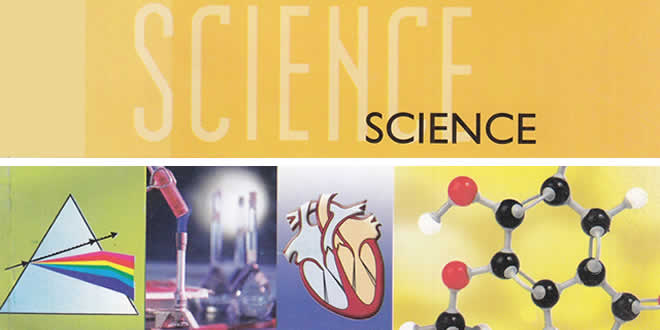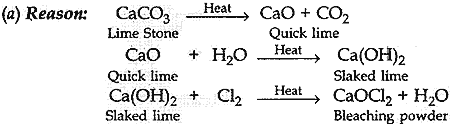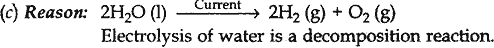Wednesday , September 28 2022# Chemical Reactions and Equations MCQs: 10 Science Ch 1

Chemical Reactions and Equations MCQs: CBSE Class 10 Science Chapter 1 Chemical Reactions and Equations Multiple Choice Questions with Answers. MCQ Questions for Class 10 Science with Answers was Prepared Based on Latest Exam Pattern. Students can solve NCERT Class 10 Science Chemical Reactions and Equations Multiple Choice Questions with Answers to know their preparation level.

 Class: 10th Class Subject: Science Chapter: Chapter 01: Chemical Reactions and Equations Quiz: 34 Questions

## Chemical Reactions and Equations MCQs

### MCQs for Class 10 Science Chemical Reactions and Equations with Answers & Explanation:

#### 1. Which of the following is a displacement reaction?

(a) MgCO3 ——-> MgO + CO2
(b) 2Na + 2H2O ——-> 2NaOH + H2
(c) 2H2 + O2 ——-> 2H2O
(d) 2Pb(NO3)2 —-Heat—-> 2PbO + 4NO2 + O2

Explanation: Reason: Here sodium (Na) displaces to form sodium hydroxide.

#### 2. Magnesium ribbon is rubbed before burning because it has a coating of

(a) basic magnesium carbonate
(b) basic magnesium oxide
(c) basic magnesium sulphide
(d) basic magnesium chloride

#### 3. Which of the following statements about the given reaction are correct?

3Fe (s) + 4H2O (g) → Fe3O4 (s) + 4 H2 (g)

(i) Iron metal is getting oxidised
(ii) Water is getting reduced
(iii) Water is acting as reducing agent
(iv) Water is acting as oxidising agent

(a) (i), (zi) and (iii)
(b) (in) and (iv)
(c) (i), (ii) and (iv)
(d) (ii) and (iv)

#### Chemical Reactions and Equations MCQs – 4. Which of the following are exothermic processes?

(i) Reaction of water with quick lime
(ii) Dilution of an acid
(iii) Evaporation of water
(iv) Sublimation of camphor (crystals)

(a) (i) and (ii)
(b) (ii) and (iii)
(c) (i) and (iv)
(d) (ii) and (iv)

Explanation: Reason: In both the cases, heat energy is evolved.

#### 5. Oxidation is a process which involves

(a) addition of oxygen
(b) addition of hydrogen
(c) removal of oxygen
(d) removal of hydrogen

#### 6. The process of reduction involves

(a) addition of oxygen
(b) addition of hydrogen
(c) removal of oxygen
(d) removal of hydrogen

#### 7. Three beakers labelled as A, B and C each containing 25 ml of water were taken. A small amount of NaOH, anhydrous CuSO4 and NaCl were added to the beakers A, B and C respectively. It was observed that there was an increase in the temperature of the solution contained in beakers A and B, whereas in case of beaker C, the temperature of the solution falls. Which one of the following statement(s) is (are) correct?

(i) In beakers A and B, exothermic process has occurred.
(ii) In beakers A and B, endothermic process has occurred.
(iii) In beaker C exothermic process has occurred.
(iv) In beaker C endothermic process has occurred.

(a) (i) only
(b) (ii) only
(c) (i) and (iv)
(d) (iv), (ii) and (iii)

(a) 1:2
(b) 1:1
(c) 2:1
(d) 1:8

#### 9. Which among the following statement(s) is (are) true? Exposure of silver chloride to sunlight for a long duration turns grey due to

(i) the formation of silver by decomposition of silver chloride
(ii) sublimation of silver chloride
(iii decomposition of chlorine gas from silver chloride
(iv) oxidation of silver chloride

(a) (i) only
(b) (i) and (iii)
(c) (ii) and (iii)
(d) (iv) only

#### 10. MnO2 + 4HCl → 2 + 2H2O + Cl2 Identify the substance oxidized in the above equation.

(a) MnCl2
(b) HCl
(c) H2O
(d) MnO2

Explanation: Reason: In this reaction HCl is oxidised to Cl2, whereas MnO2 is reduced to MnCl2.

## Chemical Reactions and Equations MCQs: 10 Science Ch 1

#### 11. A substance ‘X’ is used in white-washing and is obtained by heating limestone in the absence of air. Identify ‘X’.

(a) CaOCl2
(b) Ca (OH)2
(c) CaO
(d) CaCO3(a) AgNO3
(b) Ag2S
(c) Ag2O
(d) Ag2CO3

#### 13. Which of the following is an endothermic process?

(a) Dilution of sulphuric acid
(b) Sublimation of dry ice
(c) Condensation of water vapours
(d) Respiration in human beings

#### 14. In the double displacement reaction between aqueous potassium iodide and aqueous lead nitrate, a yellow precipitate of lead iodide is formed. While performing the activity if lead nitrate is not available, which of the following can be used in place of lead nitrate?

(a) Lead sulphate (insoluble)
(c) Ammonium nitrate
(d) Potassium sulphate

#### 15. What type of chemical reactions take place when electricity is passed through water?

(a) Displacement
(b) Combination
(c) Decomposition
(d) Double displacement

Explanation:(a) I2
(b) H2S
(C) HI
(d) S

(a) Oxidant
(b) Rancid
(c) Coolant
(d) Antioxidant

#### 18. The condition produced by aerial oxidation of fats and oils in foods marked by unpleasant smell and taste is called:

(a) antioxidation
(b) reduction
(c) rancidity
(d) corrosion

(a) 1 : 1
(b) 2 : 1
(c) 4 : 1
(d) 1 : 2

#### 20. When S02 gas is passed through saturated solution of H2S, which of the following reaction occurs?

(a) SO2 + 2H2S → 2H20 + 3S
(b) SO2 + 2H2S → H20 + 3S
(c)SO2 + H2S → H2O + S
(d) SO2 + H2O → SO3 + H2

## Chemical Reactions and Equations MCQs: 10 Science Ch 1

#### 21. Name the products formed when iron filings are heated with dilute hydrochloric acid

(a) Fe (III) chloride and water
(b) Fe (II) chloride and water
(c) Fe (II) chloride and hydrogen gas
(d) Fe (III) chloride and hydrogen gas

Explanation: Reason: 2Fe + 6HCl → 2FeCl3 (Iron (III) chloride) + 3H2

#### 22. Pb + CuCl2 → PbCl2 + Cu The above reaction is an example of:

(a) combination
(b) double displacement
(c) decomposition
(d) displacement

#### Chemical Reactions and Equations MCQs – 23. Which of the following gases can be used for storage

(a) Carbon dioxide or Oxygen
(b) Nitrogen or Oxygen
(c) Carbon dioxide or Helium
(d) Helium or Nitrogen

#### 24. A dilute ferrous sulphate solution was gradually added to the beaker containing acidified permanganate solution. The light purple colour of the solution fades and finally disappears. Which of the following is the correct explanation for the observation?

(a) KMnO4 is an oxidising agent, it oxidises FeSO4.
(b) FeSO4 acts as an oxidising agent and oxidises KMNO4.
(c) The colour disappears due to dilution; no reaction is involved.
(d) KMnO4 is an unstable compound and de-composes in presence of FeSO4 to a colorless compound.

#### 25. In which of the following chemical equations, the abbreviations represent the correct states of the reactants and products involved at reaction temperature?

(a) 2H2 (l) + O2 (l) > 2H2O(g)
(b) 2H2 (g) + O2 (l) > 2H2O (l)
(c) 2H2 (g) + O2 (g) > 2H2O (l)
(d) 2H2 (g) +O2 (g) > 2H2O (g)